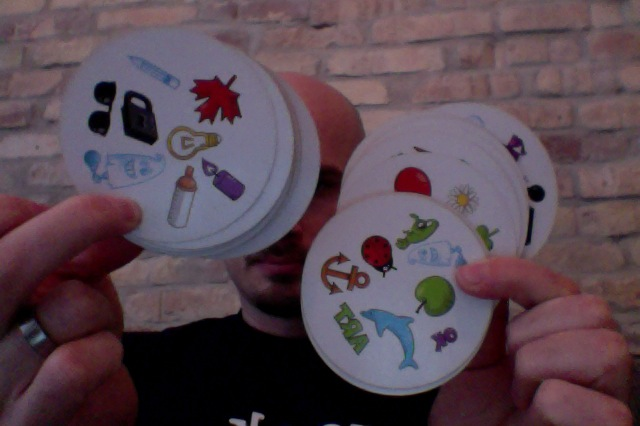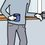# Spot It problem, version 1The game "Spot It!" consists of a deck of cards.

In the original version of the game, every card has 8 symbols on it. If you pick any two cards, exactly one symbol will be the same on the cards.

In the kids version of the game, every card has 6 symbols instead, but you still have exactly one match of symbols if you pick any two cards.

Assume that the deck contains the same number of every symbol (that is, no symbol is more common than another).

In a deck with N symbols per card:

a) How many cards will there be at most?

b) How many symbols will there be?Note by Johan Falk
6 years, 9 months ago

This discussion board is a place to discuss our Daily Challenges and the math and science related to those challenges. Explanations are more than just a solution — they should explain the steps and thinking strategies that you used to obtain the solution. Comments should further the discussion of math and science.

When posting on Brilliant:

• Use the emojis to react to an explanation, whether you're congratulating a job well done , or just really confused .
• Ask specific questions about the challenge or the steps in somebody's explanation. Well-posed questions can add a lot to the discussion, but posting "I don't understand!" doesn't help anyone.
• Try to contribute something new to the discussion, whether it is an extension, generalization or other idea related to the challenge.

MarkdownAppears as
*italics* or _italics_ italics
**bold** or __bold__ bold
- bulleted- list
• bulleted
• list
1. numbered2. list
1. numbered
2. list
Note: you must add a full line of space before and after lists for them to show up correctly
paragraph 1paragraph 2

paragraph 1

paragraph 2

[example link](https://brilliant.org)example link
> This is a quote
This is a quote
    # I indented these lines
# 4 spaces, and now they show
# up as a code block.

print "hello world"
# I indented these lines
# 4 spaces, and now they show
# up as a code block.

print "hello world"
MathAppears as
Remember to wrap math in $$ ... $$ or $ ... $ to ensure proper formatting.
2 \times 3 $2 \times 3$
2^{34} $2^{34}$
a_{i-1} $a_{i-1}$
\frac{2}{3} $\frac{2}{3}$
\sqrt{2} $\sqrt{2}$
\sum_{i=1}^3 $\sum_{i=1}^3$
\sin \theta $\sin \theta$
\boxed{123} $\boxed{123}$

Sort by:

Suppose 'm' cards have same symbol on them..

Then, for any card, we have {m-1} more cards , each bearing the N symbols. Thus, accounting for all N Symbols, it is {m-1} N cards.

Hence, No. of Cards = {m-1} N + 1

It can be proved, as per the constraint provided {all symbols occur equal times; each pair of cards have exactly one commoner} , the possible values for m are only 1 and N .

==> No. of cards {with N cards with one commoner} = [N-1] N + 1 = N^2 - N + 1

Again, for No. of symbols, leaving beside the first card, we can find that the remaining [m-1] card will have distinct symbols. The remaining symbols will repeat in the remaining cards.

No. of distinct symbols on each card in [m-1] set = N-1

No. of distinct symbols = N + [m-1] [N-1] = N + [N-1] ^2 {Since, m = N}

Thus, to conclude,

* Each symbol can repeat either twice or N times *

For one symbol repetition,

No. of Cards in the deck = N+1

No. of Symbols = N [N+1] / 2

For N Symbols repetition,

No. of Cards in the deck = N^2 - N + 1

No. of Symbols = N + [N-1] ^2

P.S: The answer I have provided is based on my understanding of the problem. In case, there is a difference, kindly notify me.

- 6 years, 9 months ago

Sweet!

I don't follow all your steps, but I follow them well enough to be convinced that this is a good solution. (I also know that the actual algebraic expressions are correct.)

Did you check out version 2 of the problem?

- 6 years, 9 months ago

Yes... It is more complicated than I thought...

I found the solutions. But, thinking of the method to derive it!!

- 6 years, 9 months ago

a) Suppose we have one card with N Symbols... We have exactly "N" more cards that has one symbol from the First Card..

Hence, No. of Cards in the Deck = N+1

b) With respect to No. of Symbols, First card has N distinct symbols. Second card has [ N-1 ] distinct symbols , while comparing to the first... Third card has [N-2]

Summing up, No. of Symbols = N + [N-1] + [N-2] + .....+1 = N [N+1] / 2

No. of Cards in the Deck = N+1

No. of Symbols = N [N+1] / 2

Edited for addition of solution for the second part of the problem.

- 6 years, 9 months ago

Nope. There may (and actually must) be more than one more card with each symbol. If card 1 has the symbol "ghost" on it, than there are more than one other card with "ghost" on it. (At least in the general case.)

- 6 years, 9 months ago

No need to have more than two cards with same symbol {even in general case}.

First card has N Symbols. Second one has 1 symbol in common with the first one. Now, if we take the third card, it is supposed to have one symbol in common with first card and, one with second card. and so on...

Hence, we can very well construct a deck of cards such that each symbol occurs only in two cards..

Let me show you that for 6 Symbols per card

Card #1: A,B,C,D,E,F

Card #2: A,G,H,I,J,K

Card #3: B,G,L,M,N,O

Card #4: C,H,L,P,Q,R

Card#5: D,I,M,P,S,T

Card #6: E,J,N,Q,S,U

Card #7: F,K,O,R,T,U

P.S: The answer I have provided is based on my understanding of the problem. In case, there is a difference, kindly notify me.

- 6 years, 9 months ago